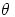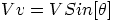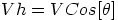## dfx's Content

There have been 189 items by dfx (Search limited from 02-December 20)

#### See this member's

Sort by                Order

### #94713vectorsPosted by on 11 March 2007 - 10:12 PM in Problem Questions

w.w = (2u+1/2v).(2u+1/2v) . The dot product is distributive so you can multiply out like you would in regular multiplication (i.e. 2u.2u = 4u.u ... etc). Note that u.u = 1 (Since Cos of zero - the angle between u and itself - is 1). u.v = v.u (the dot product is also commutative) and the value of this is given to you.

That's not quite right.

Hmm got abit carried away with the us and vs as is and js. Sorry about that.

### #94683vectorsPosted by on 10 March 2007 - 10:48 PM in Problem Questions

stuck on a few questions any help will be much appreciated

1.The triangle PQR has vertices P(1,4,-1) Q(3,4,2) and R (-2,4,1). Show that PQR is:
(i)isosceles

Isosceles implies 2 lengths are the same and 2 angles are equal if that helps.

(ii)right-angled at P

Dot product of 2 vectors extruding from the point P must be zero if they're right angled.

2.Two vectors u and v are such that magnitude of u=7, magnitude of v = 4 and u.v=14
The vector w is defined by w=2u+1/2v
Evaluate w.w and hence find the magnitude of w.

w.w = (2u+1/2v).(2u+1/2v) . The dot product is distributive so you can multiply out like you would in regular multiplication (i.e. 2u.2u = 4u.u ... etc). Note that u.u = 1 (Since Cos of zero - the angle between u and itself - is 1). u.v = v.u (the dot product is also commutative) and the value of this is given to you.

### #94661A stupid question.Posted by on 09 March 2007 - 09:36 AM in Mathematics

Out of curiosity, would it represent a circle with radius 2i?

Interesting. I'd say 'no'.

I shall agree. Thanks.### #94622Industrial chemistryPosted by on 07 March 2007 - 11:35 PM in Chemistry

Oh, yeah, I never even noticed that.

The devil is in the detail.aka typo.

### #94621A stupid question.Posted by on 07 March 2007 - 11:34 PM in Mathematics

Out of curiosity, would it represent a circle with radius 2i?

### #94607Industrial chemistryPosted by on 07 March 2007 - 08:42 AM in Chemistry

[color=#9932CC][i]After mixing 50cm cubed NaOH(aq)degrees celsius

At how many degrees celsius?

### #94338Law at Glassgow or Strathclyde.Posted by on 27 February 2007 - 07:03 AM in Course Choices

I recall someone on HSN who'd switched from Glasgow law to Strathy citing the same reasons. Can't remember who though.

### #94265Critical EssaysPosted by on 24 February 2007 - 11:30 AM in English

Try mnemonics, they always work for me. Like a short mnemonic per quote if its long. And then you just have to remember the mnemonics, which you could create another mnemonic for if they're too difficult. Rinse and repeat if necessary. But yeah, seriously, they did work for me. Hope that helps.

### #94183FRICTION!Posted by on 21 February 2007 - 04:20 PM in Physics

Try googling for "tribology", wiki links to http://www.shef.ac.uk/mecheng/tribology/te...istribology.htm which is a decent intro.

### #93824A Simple Experiment, goin not so simply!Posted by on 08 February 2007 - 10:09 PM in PhysicsIn the above formula,is known as the "angle of friction" and is the angle that the resultant of the Friction force and the Normal reaction makes with the inclined plane. But that's stretching it a little, just in case you're interested. As you can see,will vary depending on the magnitude of the friction force and traces out what's known as a "cone of friction" upto the point of slipping.

### #93798This year's paperPosted by on 08 February 2007 - 07:23 AM in Mathematics

most famous and prestigious filthy Cowley scum.

Much better.### #935003x3 MatrixPosted by on 31 January 2007 - 02:32 PM in Mathematics

What you're essentially doing when multiplying "row by column" is, if you think back to Highers, taking the dot product . Once you see it that way and you obviously are an expert at the dot product by now, it becomes much easier - well it did for me anyway.

So dot product top row by the first, second and third columns to give you the first row of your matrix: [ (2,-1,2).(3,0,-1) ....... (2,-1,2).(-1,2,-2) ...... (2,-1,2).(3,0,0) ] which gives your first row.

Similarly dot product the second (middle) row by the first, second and third columns to give your second matrix row: [ (-3,0,1).(3,0,-1) .......... (-3,0,1).(-1,2,-2) ......... (-3,0,1).(3,0,0)]

Similarly repeat for the third line.

In fact this isn't just an easy way of remembering it, it is technically true that the dot product is the product of a (1x3) matrix by its transpose, but that's getting technical and let's not do that. So two things to remember, (i) row by column and (ii) dot products. Good luck.

ps: the dots between the dot product are just there as spacers.

### #93346volume of revolution questionPosted by on 26 January 2007 - 02:24 PM in Mathematics

Work out the indefinite integral, plug in limits for t and 1 in place of x, this whole expression is equal to 40pi, and so finally solve for t. Remember you can take out the pi as a constant out of the integral sign which should make it neat. Sorry for not tex formatting guys, in a hurry.

### #93322Quantity multipliersPosted by on 25 January 2007 - 05:49 PM in Physics

You probably clicked Submit twice. Anyway, I meant for inputting data e.g. 3000 could be put in as 3 [SHIFT] K where K is in the yellow bit over a number (can't recall which one!).

### #93266mind blank...Posted by on 24 January 2007 - 02:43 PM in Mathematics

is it sin2x?

Yes.Posted by on 19 January 2007 - 02:23 PM in Mathematics

I think a link to the wolfram integrator is a must!

### #92987equilibrium ... againPosted by on 17 January 2007 - 11:21 PM in Chemistry

nope it doesn't say you are supposed to work it out some how :s

Hess's cycle, if you're supposed to work it out.

### #92787Quantity multipliersPosted by on 15 January 2007 - 10:53 AM in Physics

That's wierd lol I'm pretty sure they have it. Definitely the sim eqns anyway.### #92781Quantity multipliersPosted by on 14 January 2007 - 11:50 PM in Physics

Your typical Casio which is allowed in the exams normally has them, which is probably the best well kept secret along with the fact that they can solve simultaneous equations.

Look over your number keys and you should see a shift function for p (pico) m (milli) k (kilo) M (Mega) G (Giga) etc. Depends on what model you have of course, but most ones I've seen do.

### #92670more homework - past paper questionsPosted by on 10 January 2007 - 10:37 PM in Physics

Ah excellent, nae bother. Yeah I know I didn't really get this myself till around easter time when it clicked during a 2 week revision course if I'm honest! If you can get a 100% on radiation and matter then you'll be fine.### #92657more homework - past paper questionsPosted by on 10 January 2007 - 05:58 PM in Physics

and thank you dfx i understand it more now but why do you use u=71.7cos36 why wouldn't ouy just say u=41.7 ?

You're welcome. To the question:

71.7Cos36? Perhaps you mean 41.7Cos36, i.e. why did I use 41.7Cos36 instead of just 41.7? Well the reason is because of the way you deal with these questions: you split them into vertical components and horizontal components. Because velocity is a vector, you can resolve it into its vertical and horl components. Like John said, think about it as a right-angled triangle with 41.7 as the hypotenuse and 36 the angle between the hypotenuse and the adjacent sides. Then using ratios you can work out what either the adjacent or the opposite sides are, and that gives you the components. HSN has some really good notes that should help you.

This page goes over exactly what your question covers, and it explains it bit by bit with diagrams really well:
http://id.mind.net/~zona/mstm/physics/mech...alSolution.html

And the HSN notes:
http://www.hsn.uk.net/files/HSN61100.pdf for a basic introduction and particularly see COMPONENTS on page 6.

See also http://www.hsn.uk.net/files/HSN61200.pdf page 6 for an introduction to 2D motion, projectiles and some good problems, rather different from yours but same principles nonetheless, and quite common in the Highers.

Can I ask whether you do maths?

### #92642more homework - past paper questionsPosted by on 09 January 2007 - 11:35 PM in Physics

the question is:

the fairway on a golf course is in two horizontal parts seperated by a steep bank.
A golf ball is given an initial velocity of 41.7m/s at an angle of 36 degrees to the horizontal
The ball reaches a maximum vertical height of 19.6m above the upper fairway - the ball hits the ground at point Q (sorry cant upload the diagram) The effect of air friction on the ball may be neglected.

(a) Calculate:
(i)the horizontal component of the initial velocity of the ball;
(ii)the vertical component of the initial velocity of the ball.

so far my working is:

U=41.7m/s
V=?
A=9.8m/s²
S=19.6m
T=?

v²=u² + 2as
=41.7² + (2*9.8*19.6)
=1738.89 + 384.16
=2123.05
v =2123.05
=46.07
=46m/s

where do i go from here?

Okay for part (a) all it is asking you is to resolve the velocity into its hrl and vrtl components. For that you useandb)show that the time taken for the ball to travel from start to finish is 4.5s but i got a different answer? can someone please help me im confusedv=u + at
t=v-u/a
= 46 - 41.7/9.8
= 4.3/9.8
= 0.438
= 0.44 seconds

c)calculate the horizontal distance travelled by the ball.

Your working worries me, it shows a lack of understanding of the basics. For parabolic motion (where you shoot off something at an angle), you always consider the vertical and horizontal bits seperately. Horizontally the acceleration is zero. Vertically the acceleration is g. Note though that although they are seperate "components", you share values between them. e.g. if you know the time it takes to get from P to Q, which you may have worked out considering the vertical motion, you can use that time for finding the horizontal distance, cause logically it is the same time. Confusing? More about this later, it should become clear with the working. Read on.

Make sure you have a really good read through of your notes and understand the whole horizontal/vertical difference cause its fundamental here!

For the time taken, consider the vertical motion. The ball travels upwards to its maximum peak height, and then is instantaneously at rest, and then starts coming back down under free fall to point Q. So we can split up the vertical motion into 2 bits, one for the upward journey, one for the downward journey:

For the first bit:
You launched the golf ball at U = 41.7Sin36 (thats the vertical initial velocity), the acceleration is 9.8, final velocity is 0, you want the time.

So t = v-u / a gives you 2.5 seconds.

So this is how long the ball takes to reach the top of its parabolic path, if you imagine it half way through its flight from P to Q, it's now right at the top. It's now going to plummet downwards to Q. Again we can ignore the horizontal bit and treat the vertical motion independently.

So we know it's got a U = 0 right at the top when it comes to "rest" in mid air i.e. when it stops travelling upwards and is about to turn to come back down. It's going to accelerate freely courtesy of gravity at a = 9.8 and you also know that the vertical height it travels is s = 19.6m. Now you could use s = ut + 0.5at^2 and solve the quadratic for t, but that's complicating things. Instead use v^2 = u^2 + 2as and solve for V the final vertical velocity as it's coming back down and about to hit point Q. If you solve this you end up with V = 19.6. Then you know V, U, a and you need the time t, which ends up nicely as 2 seconds.

So the total time is 2.5 + 2 = 4.5s

For the total distance travelled, we'll look at the horizontal component since the distance is horizontal too!

So we know U = 41.7Cos36

We also know it cannot accelerate horizontally (the reason we consider acceleration vertically is obviously cause g acts the whole time, but horizontally there's no force pushing it or stopping it, so will remain at a constant velocity.... and that's the bottom line cause Newton said so). Therefore, a = 0.

We also know the time it takes to get from P to Q, because we worked that out earlier. This was the confusing bit I was on about, but I hope it makes sense now. If you chuck something half way accross the room (on a parabolic path i.e. launch it at an angle), the time it takes to get to the top of its motion and back down is obviously the total time for the horizontal bit too!

So you know the time, the velocity and since a = 0, you can work out s = vt where v is the horizontal component 41.7Cos36.

Trajectories are abit tricky but once you get it all, it's very intuitive. Just read your stuff and ask any questions you may have.

another question is:

a student performs an experiment to study the motion of the school lift as it moves upwards
the student stands on the bathroom scales during the lift's journey upwards
the student records the reading on the scales at different parts of the lift's journey as follows:

part of journey - reading on the scales

At the start(while the lift is accelerating) - 678N
In the middle (while the lift is moving at a steady speed) - 588N
At the end (while the lift is decelerating) - 498N

(a)show that the mass of the student is 60kg
(b)calculate the initial acceleration of the lift
©calculate the deceleration of the lift
(d) during the journey, the lift accelerates for 1.0s, moves at a steady speed for 3.0s and decelerates for a further 1.0s before coming to rest. sketch the acceleration - time graph fot this journey

i think i am able to do part (b)© and (d) by myself

I would be very grateful if anyone could give me any help.

For part (a), look at it this way. What are the forces acting on the bathroom scales? You have the weight of the student, and the pushing/pulling/upthrust force of the lift. So you are given 3 sets of forces, and all you can use is F = ma. But you don't know the accelerations associated with these forces. What you do know, however, is that when it is moving at a steady speed (i.e. constant velocity, no acceleration, so no extra force there to make it accelerate), the only force acting on the scales will be the students weight and the upthrust of the lift, which is equal to the weight cause there is no acceleration either upwards or downwards. So 588 = mg, solve for m to get 60Kg. Hope that helps.### #92639homework - past paper questionsPosted by on 09 January 2007 - 11:12 PM in Physics

for (i) i got 98m so for the displacement in (ii) do i double the displacement from (i) to make s=196m ?

Yes, that's correct, as it says s is doubled.

Christ, I have a B in Higher Physics and have no idea what you said lol, SUVAT?

Evidently not.### #92594homework - past paper questionsPosted by on 09 January 2007 - 05:36 PM in Physics

i) SUVAT equations, straightforward plugging in of the values, solve for S.
ii) Knowing S, SUVAT again and solve for V.

Hold on that that V, let's call it Vii. Go back to (i) and solve V this time, and let's call the V from (i) um how about Vi. That gives you your 2 final velocities for the 2 situations, subtract Vi from Vii and you get the amount by which Vii is greater.

(iii) SUVAT is today's magic word yet again. You know your u, your v is zero, given a (remember its a negative value), solve for s.

Good luck and post back if that's vague, but it is reasonably straightforward.### #92474Conditional/Uncondtional Offers from UniversitiesPosted by on 04 January 2007 - 01:44 AM in Course Choices

OH YES!Good man! Always knew you'd get one. College?
ThaniksI went for Emmanuel, mainly for the ducks. It's John's you are at, right?

Yep. Werlop's at Emma too I believe. I gotta say though, 1,1 in Step is a bitch. But then again Emma's the pretty hardworking type so I guess it prepares you for it. Good luck!